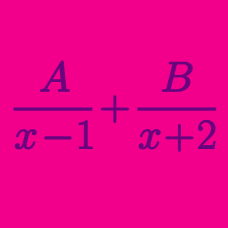Algebra

# Partial Fractions - Repeated Factors

If the following is an identity in $$x$$: $\frac{4x^2+27x+33}{(x-1)(x+3)^2}=\frac{a}{x-1}+\frac{bx+c}{(x+3)^2},$ what is the value of $$a+b+c$$?

Suppose $$a$$, $$b$$, and $$c$$ are constants such that the following holds for all real numbers $$x$$ such that all of the denominators are nonzero:

$\frac{9}{x(x+1)^2}=\frac{a}{x}+\frac{b}{x+1}+\frac{c}{(x+1)^2}.$ What is the value of $$abc?$$

If the following is an identity in $$x$$: $\frac{17x^3+7x^2-17x-7}{(x-1)^2(x+1)^2}=\frac{A}{x-1}+\frac{B}{x+1},$ what is the value of $$A \times B?$$

If the following is an identity in $$x$$: $\frac{11x^2+90x+99}{(x-3)^2(x+3)^2}=\frac{A}{(x-3)^2}-\frac{B}{(x+3)^2},$ what is the value of $$A \times B?$$

If the following is an identity in $$x$$: $\frac{-13x+32}{(x+1)(x-2)^2}=\frac{a}{x+1}+ \frac{ b} { (x-2) } + \frac{ c} { (x-2)^2} ,$ what is the value of $$a+b+c$$?

×

Problem Loading...

Note Loading...

Set Loading...